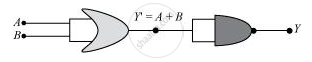# The Output of an Or Gate is Connected to Both the Inputs of a Nand Gate Draw the Logic Circuit of this Combinaion of Getes and Write Its Truth Table. - Physics

#### Question

The output of an OR gate is connected to both the inputs of a NAND gate Draw the logic circuit of this combinaion of getes and write its truth table.

#### Solution 1

Both the inputs of the NAND gates are joined to form a single input. Therefore, it behaves like a NOT gate.A B A + B overline(A+B) 0 0 0 1 0 1 1 0 1 0 1 0 1 1 1 0

#### Solution 2

Both the inputs of the NAND gates are joined to form a single input. Therefore, it behaves like a NOT gate.A B A + B overline(A+B) 0 0 0 1 0 1 1 0 1 0 1 0 1 1 1 0

Is there an error in this question or solution?

#### Video TutorialsVIEW ALL 

The Output of an Or Gate is Connected to Both the Inputs of a Nand Gate Draw the Logic Circuit of this Combinaion of Getes and Write Its Truth Table. Concept: Digital Electronics and Logic Gates.